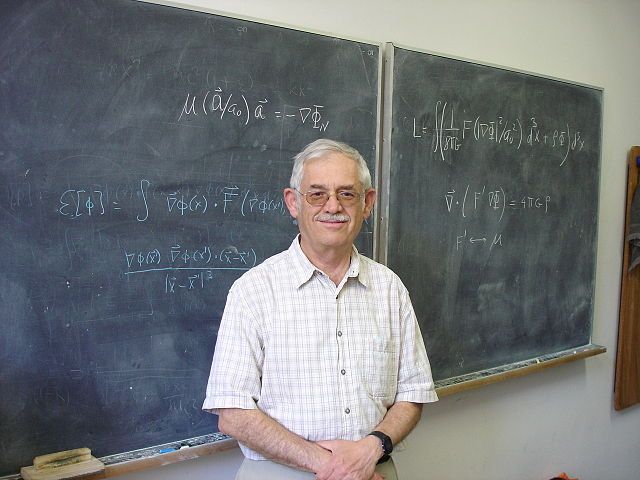I woke up this morning to the news that Mexican-Israeli physicist Jacob Bekenstein died two days ago, at the age of 68, in Helsinki, Finland. I saw nothing about the cause of death.Bekenstein’s work is well known to folks dealing with gravity theory. Two of his contributions stand out in particular.

First, Bekenstein was first to suggest that black holes should have entropy. His work, along with that of Stephen Hawking, led to the Bekenstein-Hawking entropy formula $$S=kc^3A/4G\hbar$$, relating the black hole’s surface area $$A$$ to its entropy $$S$$ using the speed of light $$c$$, the gravitational constant $$G$$, the reduced Planck constant $$\hbar$$ and Boltzmann’s constant $$k$$. With this work, the science of black hole thermodynamics was born, leading to all kinds of questions about the nature of black holes and the connection between thermodynamics and gravity, many of which remain unanswered to this day.

Bekenstein’s second contribution was to turn Morehai Milgrom’s MOdified Newtonian Dynamics (MOND) into a respectable relativitistic theory. The MOND paradigm is about replacing Newton’s law relating force $$({\mathbf F})$$, mass $$(m)$$ and acceleration $$({\mathbf a})$$, $${\mathbf F}=m{\mathbf a}$$, with the modified law $${\mathbf F}=\mu(a/a_0)m{\mathbf a}$$, where all we know about the function $$\mu(x)$$ is that $$\lim_{x\to 0}\mu(x)=x$$ and $$\lim_{x\to\infty}\mu(x)=1$$. Surprisingly, the right choice of $$a_0$$ results in an acceleration law that explains the anomalous rotation of galaxies without the need for dark matter. However, in this form, MOND is theoretically ugly: it is a formula that violates basic conservation laws, including the consevation of energy, for instance. Bekenstein’s TeVeS (Tensor-Vector-Scalar) gravity theory provides a general relativistic framework for MOND, one that does respect basic conservation laws, yet reproduces the MOND acceleration formula in the low energy limit.

I never met Jacob Bekenstein, and now I never will. A pity. May he rest in peace.